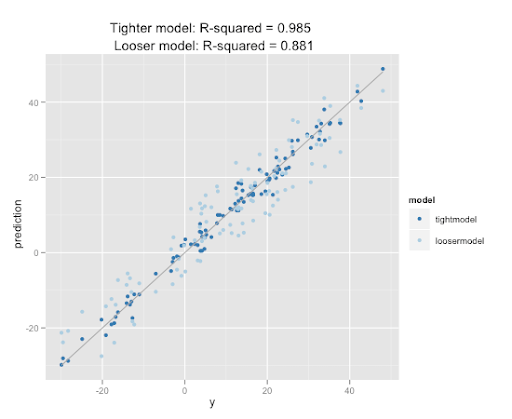top of page

# Intro to R²

### By Jasmine Shone

If you’ve ever been faced with the task of defining the validity of a regression model, you’ve probably heard of R². But do you know how exactly R² is defined, its pitfalls, and alternative forms that attempt to correct those shortcomings? If not, or if you need some review, read on.How R² describes model performance. Image Source: https://www.quora.com/what-is-the-difference-between-r-and-R-squared

You’ve probably heard about vanilla R² at some point if you are interested in machine learning and statistics. The formula for r² is

1- (sum of squares of residuals)/(total sum of squares)

Where residuals are the “offset” of the variable you want to predict from each data point(target variable) from the model prediction. For example, if the predicted outcome is 10 and the real value is 8, the residual would be -2.

The “squares” are the target variable values with the mean of the target variable subtracted. For example, if you are predicting the temperature in Miami and the average of the temperature data is 80 degrees Fahrenheit, then you would perform (value - 80)² for each value of temperature then add them together.

Through this step, you compare your model to the theoretically worst model, a straight line at the mean. The smaller the residuals from your model are, the smaller the fraction ((sum of squares of residuals)/(total sum of squares)) is and the greater the overall equation (1- (sum of squares of residuals)/(total sum of squares)) becomes.

• For linear regression (i.e. Fitting a straight line), R² is used pretty often.

• R² can also be more easily interpretable than error metrics. For example, if your MAE is 0.3, the meaning is less clear than if you get an R² of 0.7, to which you can state that 70% of the variance in the target variable is explained by the model.

• R² is considered a biased estimator, meaning that in a sample R² is systematically higher than in the population.

• A high R² could indicate overfitting. Overfitting happens when the model describes random variation/noise in the sample instead of focusing on the significant relationships between variables.

• R² tends to increase when more variables are added to the model. This could lead to makers of a model continuously adding variables even if they are not beneficial or make predictions on other samples from the overall population worse.

The AlternativesEven if additional variables are random noise, R² continues to increase. Adjusted R² does not. Image Source: https://r4all.org/posts/why-adjust-your-r-squared/

One of the shortcomings of vanilla R² that is especially negative when developing a model is its bias towards adding more variables. Adjusted R² attempts to correct for this by taking into account the number of features used to predict the target variable. The formula is:

1-[(1-R²)* (n-1)/(n-k-1)]

Where R² is the regular R² value, n is the number of data points, and k is the number of independent variables. In this case, if k increases while R² stays the same, the overall adjusted R² value will decrease.

Predicted R²R² and R² (adj) could be significant while R² (predicted) is not; this mostly happens when the model overfits the data. Image Source: https://blog.minitab.com/en/adventures-in-statistics-2/multiple-regession-analysis-use-adjusted-r-squared-and-predicted-r-squared-to-include-the-correct-number-of-variables

Predicted R² attempts to address the problem of overfitting in vanilla R² through an approach very similar to cross validation. To calculate predicted R², you fit the model with the variables you chose on all of the data points except for one. Then, you evaluate how well the model predicts the missing data point, and repeat over every data point.

Due to its ability to indicate overfitting, predicted R² is a very valuable tool to supplement the other R²s, given that neither account for this problem.

Summary

R² remains a very popular validation metric for regression. Unfortunately, vanilla R² is a metric with many shortcomings, some of which is remedied by predicted or adjusted R². So next time you think about using R², you should consider using the other two forms of R² to supplement your evaluation, or choose another metric altogether.

1 comment

See All
bottom of page